#### WATCH ALL SLIDES

Slide 1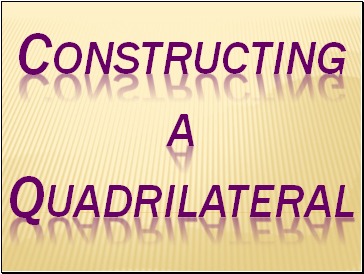Slide 2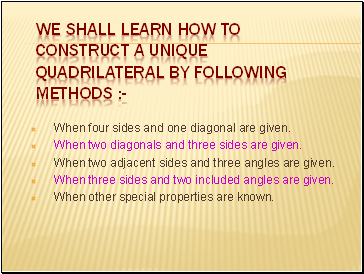We shall learn how to construct a unique quadrilateral by following methods :-

When four sides and one diagonal are given.

When two diagonals and three sides are given.

When two adjacent sides and three angles are given.

When three sides and two included angles are given.

When other special properties are known.

Slide 3Constructing a quadrilateral when four sides and one diagonal are given

Example : Construct a quadrilateral PQRS where PQ = 4cm, QR = 6cm, RS= 5cm, PS = 5.5cm and PR = 7cm

7 cm

4 cm

6 cm

5.5 cm

5 cm

S

R

Q

P

ROUGH SKETCH

Slide 4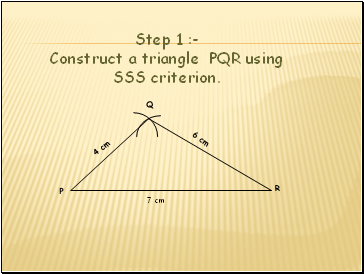4 cm

6 cm

R

P Step 1 :- Construct a triangle PQR using SSS criterion.

7 cm

Q

Slide 5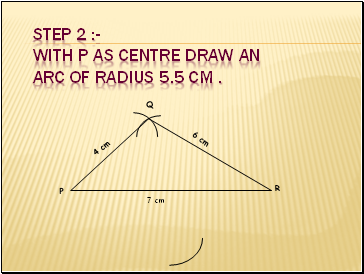Step 2 :- With P as centre draw an arc of radius 5.5 cm .

4 cm

6 cm

R

P

7 cm

Q

Slide 6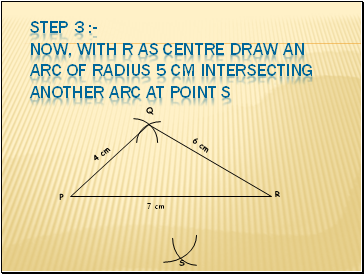Step 3 :- Now, with R as centre draw an arc of radius 5 cm intersecting another arc at point S

4 cm

6 cm

R

P

7 cm

Q

S

Slide 7Step 4 :- Now join PS and RS.

4 cm

6 cm

S

P

7 cm

Q

R

Slide 8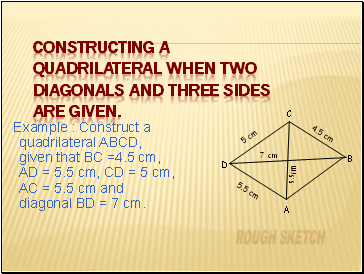## Constructing a quadrilateral when two diagonals and three sides are given.

Example : Construct a quadrilateral ABCD, given that BC =4.5 cm, AD = 5.5 cm, CD = 5 cm, AC = 5.5 cm and diagonal BD = 7 cm.

ROUGH SKETCH

C

D

A

B

7 cm

5 cm

5.5 cm

5.5cm

4.5 cm

Slide 9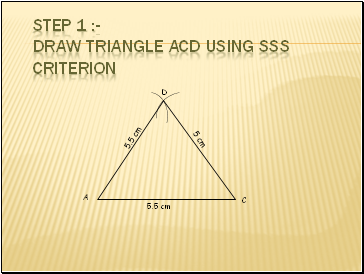Step 1 :- Draw triangle ACD using SSS criterion

C

D

A

5.5 cm

5 cm

5.5 cm

Slide 10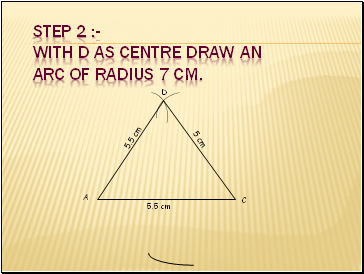Step 2 :- With D as centre draw an arc of radius 7 cm.

C

D

A

5.5 cm

5 cm

5.5 cm

Slide 11Step 3 :- Now, with C as centre draw an arc of radius 4.5 cm intersecting another arc at point B.

C

D

A

5.5 cm

5 cm

5.5 cm

B

Slide 12Go to page:
1  2  3